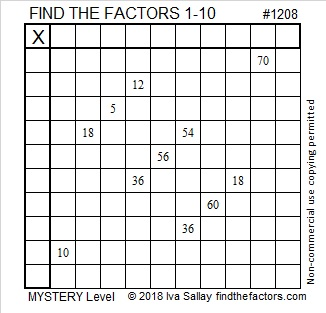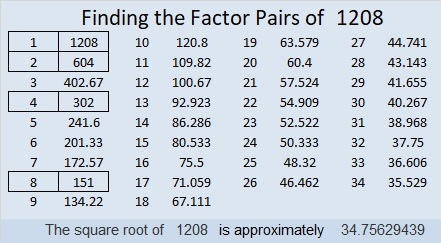# 1208 Mystery Level

The factors in the multiplication table puzzle below are not in the usual order. Can you figure out where each factor from 1 to 10 belongs in both the first column and the top row?Print the puzzles or type the solution in this excel file: 10-factors-1199-1210

Here are a few facts about the number 1208:

• 1208 is a composite number.
• Prime factorization: 1208 = 2 × 2 × 2 × 151, which can be written 1208 = 2³ × 151
• The exponents in the prime factorization are 3 and 1. Adding one to each and multiplying we get (3 + 1)(1 + 1) = 4 × 2 = 8. Therefore 1208 has exactly 8 factors.
• Factors of 1208: 1, 2, 4, 8, 151, 302, 604, 1208
• Factor pairs: 1208 = 1 × 1208, 2 × 604, 4 × 302, or 8 × 151
• Taking the factor pair with the largest square number factor, we get √1208 = (√4)(√302) = 2√302 ≈ 34.756291208 is also the sum of consecutive prime numbers:
601 + 607 = 1208